This blog is managed by Song Hock Chye, author of Improve Your Thinking Skills in Maths (P1-P3 series), which is published and distributed by EPH.

## Monday, February 25, 2008

### Are we seeing a change in role of teachers in schools?

A teacher's viewpoint on the teaching profession today.

Am I Now A Researcher Too?

Looks like even teachers today are expected to adapt to changes. Gone are the days when civil servants can expect the same pay, same job, for the rest of their careers.

### Water freezes below 0 deg Celsius

Interesting video showing water freezing in Newfoundland, Canada. The waves freeze upon impact!

## Wednesday, February 20, 2008

### Interesting viewpoint on the competition in schools from a school teacher

Here is an article I found that points to the fact that it is getting more and more difficult to get into top schools - Inflation in Schools.

### Tao Nan School P6 Math CA1 2004 (Q49)

The tank below was 30% filled with water. Taps A and B were turned on at the same time. Water flowed from Tap A at the rate of 196 cubic cm per second, while Tap B at 100 cubic cm per second.

a) Find the volume of water in the tank before the taps were turned on.
b) How much time was needed to fill 40% of the tank?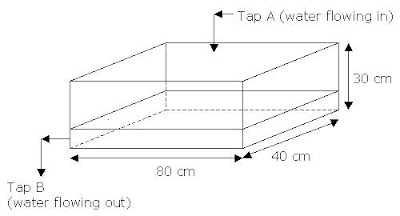Solution

Q(a)

Water was filled up to 30% of the tank. Therefore volume of water in the tank is

80 cm x 40 cm x 30 cm x 30%
= 28 800 cubic cm
= 28.8 litres

Answer: The volume of water in the tank before both taps were turned on was 28.8 litres.

Q(b)

For the tank to be filled up to 40%, water is needed to fill it up by another 10% of the tank.

30% of the volume of the tank holds 28.8 litres.
10% will hold ----- 28.8 litres divided by 3 = 9.6 litres

Tap A had a rate of 196 ml per second, while Tap B 100 ml per second.

Net flow of water into the tank is therefore 196 ml per sec – 100 ml per sec = 96 ml per sec.

Time taken to fill up 9.6 litres -----
9.6 litres divided by 96 ml per sec
= 100 seconds
= 1 min 40 seconds.

Answer: It takes 1 min 40 seconds to fill up to 40% of the tank.

## Monday, February 18, 2008

### Tao Nan School P6 Math CA1 2004 (Q47)

A farmer had 400 animals. 20% of them were cows and the rest were goats. He bought some more cows and goats. The number of goats increased by 20% and the number of cows increased by 40%. How many animals did he buy altogether?

Solution

Before more cows and goats were bought

Cows ----- 20% x 400 (animals) = 80

Goats ----- 80% x 400 (animals) = 320

After more cows and goats were bought

Cows ----- 80 x 40% = 32

Goats ----- 320 x 20% = 64

Total animals bought 32 + 64 = 96

Answer: He bought 96 animals altogether.

## Thursday, February 14, 2008

### Tao Nan School P6 Math CA1 2004 (Q46)

A skirt and 3 blouses cost \$78. The skirt costs \$p more than a blouse.
a) Express the cost of a blouse in term of p.
b) If p=10, how much is the cost of the skirt?

Solution

Skirt ----- 1 unit + \$p
Blouse ----- 1 unit

Q(a)
Skirt + 3 blouses ----- \$78
(1 unit + \$p) + (3 units) ----- \$78
4 units + \$p ----- \$78
4 units ------ \$78 - \$p
1 unit ----- \$(78 – p)/4

Answer: The cost of a blouse is \$(78 – p)/4

Q(b)
1 unit ----- \$(78 – 10)/4 = \$17

Skirt ----- 1 unit + p
= \$17 + \$10 = \$27

Answer: The cost of the skirt is \$27

## Tuesday, February 12, 2008

### Tao Nan School P6 Math CA1 2004 (Q45)

Carol’s salary is 3/8 of Ben’s salary. David’s salary is 5/6 of Carol’s. After Ben gives \$70 to David, David has twice as much money as Carol.
a) How much does David have now?
b) What is the new ratio of David’s salary to Carol’s salary to Ben’s salary? (Express your answer in the simplest form)

Solution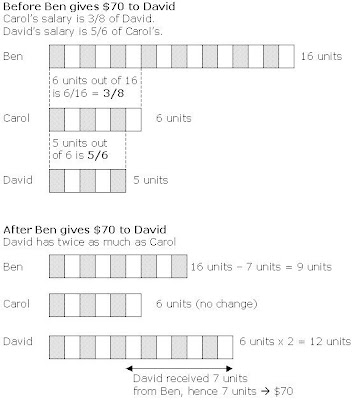7 units ----- \$70
1 unit ----- \$70 divided by 7 = \$10

Q(a) David now has

12 units ----- \$10 x 12 = \$120

Q(b)Answer: The new ratio of David’s salary to Carol’s salary to Ben’s salary is 4 : 2 : 3

## Monday, February 11, 2008

### Tao Nan School P6 Math CA1 2004 (Q44)

The figure below is made up of 2 rectangles and a square. Its dimensions are given in cm.

a) Find the perimeter of the figure in terms of n.
b) Find the area of the figure in terms of n.Solution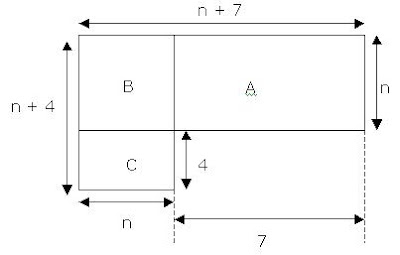Q(a)

Perimeter is (n + 7) cm + n cm + 7 cm + 4 cm + n cm + (n + 4) cm = (4n + 22) cm## Tuesday, February 05, 2008

### Tao Nan School P6 Math CA1 2004 (Q43)

Ravi and Ali shared a sum of money in the ratio of 4 : 7. After Ravi spent ¼ of his money, he had \$32 less than Ali.
a) How much money had Ravi left?
b) Ali then spent ½ of his money. What is the new ratio of the amount of money Ravi has to the amount of money Ali has?

Solution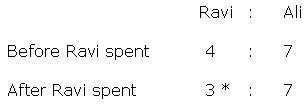* Ravi spent ¼ of his money. ¼ of 4 units is 1 unit. Therefore after spending, Ravi would have 3 units left.

The difference between Ali and Ravi after Ravi spent ¼ of his money is

7 units (of Ali’s money) – 3 units (of Ravi’s money) = 4 units

4 units ---- \$32 (Ravi had \$32 less than Ali)

1 unit ----- \$32 divided by 4 = \$8

Q(a)

3 units ----- \$8 x 3 = \$24.

Q(b)

Ali then spent half of his money. Since Ali had 7 units, half of that would mean 3 and a half units spent.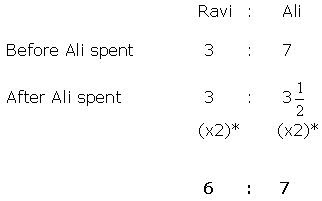* Multiply by 2 to give a whole number for Ali’s ratio of 3½.

Answer: The Ratio of amount of money Ravi has to the amount of Ali has is 6 : 7.

## Sunday, February 03, 2008

### Math Question on Area

The diagram below is made up of 3 squares. Find the area of the shaded area.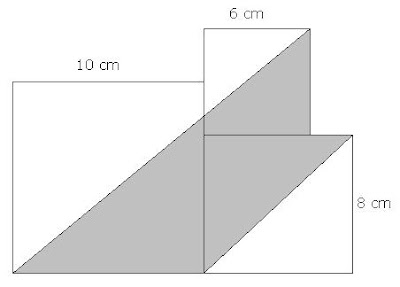SolutionArea of triangle ADC – Area of triangle BGC + Area of triangle EFG

½ x 16cm x 14cm = 112 square cm

Area of triangle BGC
CG = 6 cm because EG = EF = 2 cm
Therefore area = ½ x 6cm x 6cm = 18 square cm

Area of triangle EFG
½ x 2cm x 2cm = 2 square cm

Therefore area of shaded area is
(112 – 18 + 2) square cm

## Friday, February 01, 2008

### Tao Nan School P6 Math CA1 2004 (Q40)

Mrs Ang bought 44 cakes. The ratio of the number of butter cakes to the number of chocolate cakes was 6 : 5. After giving some chocolate cakes to her neighbours, Mrs Ang had twice as many butter cakes as chocolate cakes. How many chocolate cakes did she give away?

SolutionAfter giving away some cakes, she had twice as many butter cakes as chocolate cakes.

Since no butter cakes were given, the number of units of butter cakes remains as 6.

* Half of 6 units will give 3 units of chocolate cakes left, after some of them have been given away.

This means that 2 units of chocolate cakes have been given away.

1 unit ----- 4 cakes

2 units ----- 8 cakes

Answer: She gave away 8 chocolate cakes.